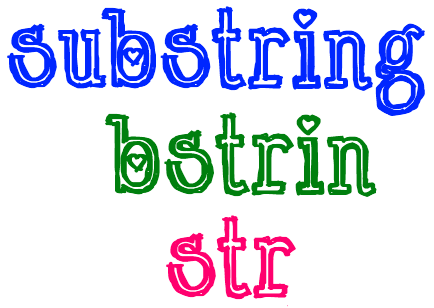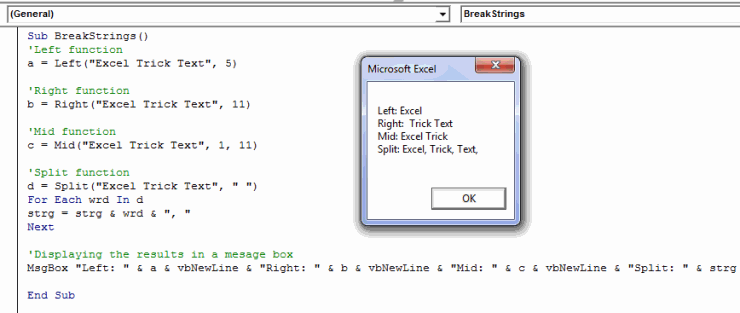# VBA Substring – How to Substring in Excel Macro

Substring is one of the most popular functions in any programming language. It eases your tasks while dealing with strings. As the name suggests a substring function divides a string into different parts based on particular criteria.

There are multiple VBA Substring functions. In practical situations, these substring functions can be quite useful in extracting a portion of a string.

Today in this post I am going to explain all the VBA substring functions that you can use in Excel macros:## LEFT Substring function:

The LEFT function in Excel VBA is used for fetching a specified number of characters from the start of the string. The syntax of the LEFT function is as follows:

`Left (text_string, length)`
• Here ‘text_string’ refers to an input string that is to be separated.
• And ‘length’ refers to the number of characters to be extracted.

### Examples:

`Left ("Exceltrick", 5) 'gives an output "Excel"Left ("SomeText", 4) 'gives the result "Some"`

Note: Instead of using a hardcoded string in the first argument you can also fetch ‘text_string’ from your excel sheet like ActiveSheet.Range(“A1”).

## Right Substring function:

The RIGHT Function in Excel VBA is just opposite to the LEFT function. It returns a specified number of characters from the end of the text string. The syntax of the RIGHT function is as follows:

`Right (text_string, length)`
• Here ‘text_string’ refers to an input string that is to be separated.
• And ‘length’ refers to the number of characters to be extracted but extraction begins from the right side.

### Example:

`Right ("Exceltrick", 5) 'gives an output "trick"Right ("SomeText", 4) 'gives the result "Text"`

Note: Instead of using a hardcoded string in the first argument you can also fetch ‘text_string’ from your excel sheet as ActiveSheet.Range(“A1”).

## MID Substring function:

MID is a much better function than the first two, it gives you the ability to specify the start and end positions of the extracted string. The syntax of the MID VBA Substring function is as under:

`Mid(text_string, start_position, Length)`
• Here ‘text_string’ refers to an input string that is to be separated.
• ‘start_position’ refers to the numeric position from where extraction is to be started.
• And ‘length’ refers to the number of characters to be extracted.

### Example:

`MID ("Exceltrick", 2,4) 'gives an output "celt"MID ("SomeText", 4,4) 'gives the result "Text"`

Note: Instead of using a hardcoded string in the first argument you can also fetch ‘text_string’ from your excel sheet as ActiveSheet.Range(“A1”).

## SPLIT Substring function:

The SPLIT function is another VBA function that can be used for sub-stringing or splitting a string. The SPLIT function can come very handy when you are dividing a text string into more than one parts based on a delimiter. The syntax of a split function is as under:

`Split (text_string, Delimiter, limit, Compare)`
• Here, ‘text_string’ refers to an input string that is to be separated.
• ‘Delimiter’ refers to the delimiter character which separates the string into parts. This is an optional argument, if it is left blank then, the space character ” ” is assumed to be the default delimiter.
• ‘limit’ refers to the maximum number of substring parts into which the string should be divided. It is also an optional argument, the default value is (-1) which means that substring should happen at every position where the delimiter is encountered.
• ‘compare’ is an optional numerical value that specifies the comparison to use when evaluating substrings.

### Example:

For instance, you have a text string as “This is a text string” and now you have to break this string into individual words, so in this case, you will use space ” ” as a delimiter. The split function will be used as:

`Split ("This is a text string", " ")`

The result of this split function is an array of words: “This” “is” “a” “text” “string”.

## VBA Substring Macro Example:

Below I have created a macro that illustrates all the substring techniques available in VBA programming. This is a simple and self-explanatory macro, in this, I have simply divided a text string with the 4 methods that I have described above.Below is the code that I have used for this macro:

`Sub BreakStrings()'Left functiona = Left("Excel Trick Text", 5)'Right functionb = Right("Excel Trick Text", 11)'Mid functionc = Mid("Excel Trick Text", 1, 11)'Split functiond = Split("Excel Trick Text", " ")For Each wrd In dstrg = strg & wrd & ", "Next'Displaying the results in a mesage boxMsgBox "Left: " & a & vbNewLine & "Right: " & b & vbNewLine & "Mid: " & c & vbNewLine & "Split: " & strgEnd Sub`

So, this was all about VBA substring functions. Do share your view related to the topic.About Ankit Kaul

Ankit is the founder of Excel Trick. He is tech Geek who loves to sit in front of his square headed girlfriend (his PC) all day long. :D. Ankit has a strong passion for learning Microsoft Excel. His only aim is to turn you guys into 'Excel Geeks'.

1.rasheedsays
•Ankit Kaulsays